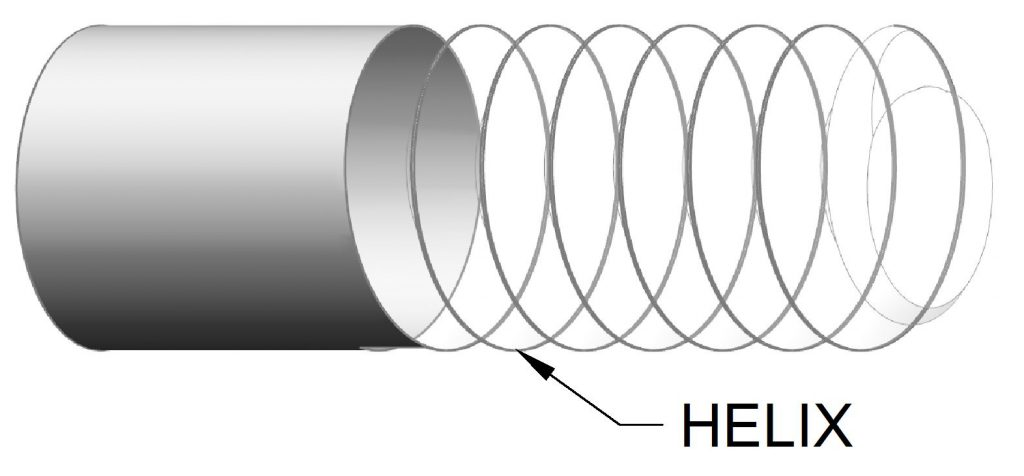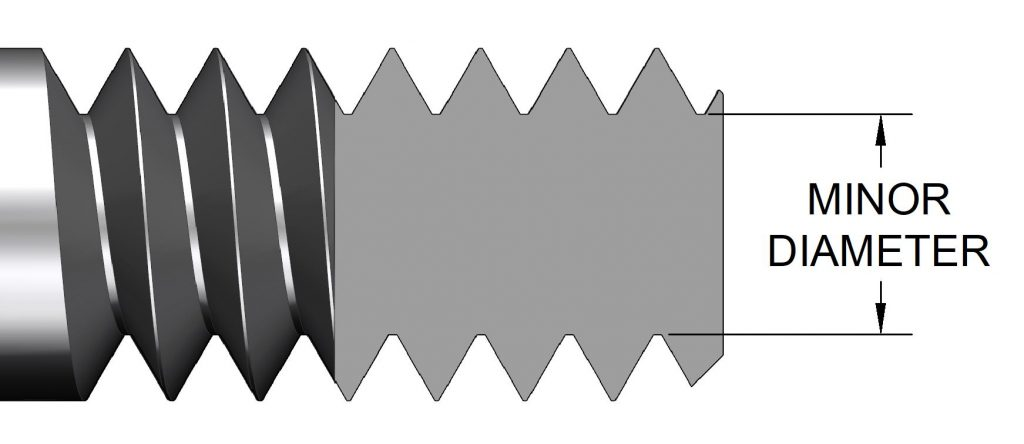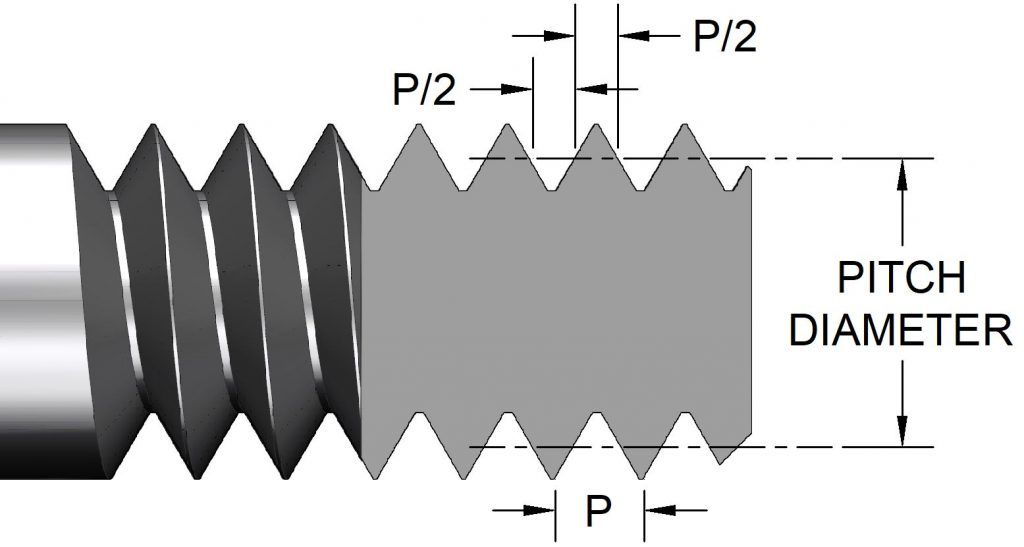## Allowance

Allowance is the difference between the maximum size of the thread and the basic size. It is usually a gap that functions as clearance but can be interference (as with NC thread forms).

## Basic Thread Profile

The basic thread profile is the boundary between the geometry that defines the internal thread and the external thread. It is a theoretically perfect form that establishes both tolerance zones. See illustration at allowance.

## Chamfer

Chamfer is a conical surface a the end of a thread. The chamfer allows easy engagement of threaded parts and prevents the formation of a sharp edge on the first thread.

## Crest

The crest is the top of the thread.

See height.

## Flank

The flank is the side of the thread. It connects the root and crest and becomes the mating surface on an assembled thread. There are two flanks on a thread, the leading flank and the following flank. The leading flank is the one that goes into the threaded hole first.

## Flank Angle

The flank angle is the angle formed between the the flank and an axis perpendicular to the axis of the thread. On symmetrical threads the flank angle is equal to half of the thread angle.The flank angle is the angle between the flank and an axis perpendicular to the thread axis.

## Form

The form of a thread is the shape of it’s profile in cross-section. See Thread Forms main article.

## Height

The height (or depth) of a thread is the distance between the root and crest of a thread.

## Helix

The curve formed on a cylinder by a straight at an angle to the axis that is wrapped around that cylinder.The helix is a straight line wrapped around a cylinder.

## Helix Angle

The helix angle is the complement of the lead angle. See illustration at lead angle.

Lead is the distance a thread will move axially in one turn. On threads with one start it is equal to the pitch. See illustration at lead angle. See also starts.

The lead angle is the angle between the helix of a thread and a plane perpendicular to the axis.

## Major Diameter

The major diameter is the diameter of the bounding cylinder of the largest dimension of the thread. It is the diameter of the crests of the threads on an external thread. The basic major diameter is the diameter of the basic thread form profile. See illustration at allowance.The major diameter is the diameter of the outside of an external thread.

## Minor Diameter

The minor diameter is the diameter of the cylinder bounded by the roots of the threads of an external thread or the crests of an internal thread.The minor diameter is the diameter of the smallest bounding cylinder of a thread.

## Pitch

The pitch is the distance between corresponding points on adjacent threads. It is the distance from one thread to the next. On a thread with one start, the pitch equals the lead.

## Pitch Diameter

The pitch diameter is the diameter of an imaginary cylinder that exactly divides the thread and groove equally. The pitch diameter is an important dimension for mating threads.The pitch diameter is the diameter of cylinder that divides thread and groove equally.

## Root

The root of a thread connects flanks of adjacent threads. It is the bottom of the thread.

## Starts

It is possible to have more than one thread on a threaded part. These multiple, individual threads are referred to as “starts.”

Notice on the three-start thread below that the lead is three times longer than the pitch.